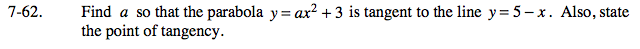### Home > CALC > Chapter 7 > Lesson 7.2.1 > Problem7-62

7-62.At the point of tangency ax2 + 3 = 5 − x

Since the tangent line is y = 5 − x, then the slope of the tangent is −1 at the point of tangency.
Therefore 2ax = −1.

Solve a system of equations.